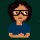Coffee Room
Discuss anything here - everything that you wish to discuss with fellow engineers.
12913 Members
Join this group to post and comment.# Is zero an even no or odd no?

Today i was studying Group theory..so this question arises in my mind
What do you think guys...zero an even no or odd no?Generally Even number is called an integer which is exactly divisible by 2.

So even numbers are considered to be in the form of 2k.

so zero is an even number.

Check this link for more detailed answer.

Evenness of zero - Wikipedia, the free encyclopediacooltwins • Dec 23, 2009
yeah even nos. are those which can be divided by 2 so zero falls under the even category
also only if x%2=1 the no. is considered odd and 0%2=0 so it is even
besides it is generally oddno.,even no., oddno.,even no., oddno.,even no.,... order so the no. before an odd no. i.e., 1 is undoubtedly evenEddy boy • Dec 23, 2009
zero is even if you think it is odd than give the characteristic of zero that matches with odd one....zero is unique digit in number system
...thanksHere is the answer which i got from a website
Zero is an even number. An integer n is called *even* if there exists
an integer m such that n = 2m, and *odd* if n+1 is even. From this,
it is clear that 0 = (2)(0) is even.Guttu • Dec 23, 2009
Zero doesn't exists. So it cannot be odd or even.durga ch • Dec 23, 2009
i go with Guttu.
Zero indicates emptiness. So how do you classify something which doesnot exists?
I guess this very emptiness gave rise to the principle of infinitness i.e 2/0 = infinity.

yes, if you consider zero as a number, the above justification works stating 0/2 et al make sense.MaRo • Dec 23, 2009
Zero indicates the emptiness that gives a high value when attached to a non-empty value, so emptiness itself is a value, if there's no emptiness there wouldn't be more.

P.S.: just a thought not sure about its validity.skipper • Dec 23, 2009
Factorial 0 is 1. Then there is some argument about 0[sup]0[/sup].
Zero is a quotient group in the integers as Z/nZ, n = 0, a modular form equivalent to div 0.

Div 0 means "divide zero times", not "divide by zero".Yep I go with skipper here!!vik001ind • Dec 24, 2009
0 in non positive & non negative integer. By the definition of evenness & oddness, any no divisible by 2 is even & otherwise odd.
So 0 is even , as 0/2 = 0 & 0 is a whole no.callyD • Jan 3, 2010
I second vik001ind's comment... Zero is an even number, as 0 is divisible by 2, and leaves no remainder. 0 is neither positive nor negative.